Mathematical Problems For the Next Century
Steve Smale

Department of Mathematics • City University of Hong Kong • Kowloon, Hong Kong • August

Introduction. V. I. Arnold, on behalf of the International Mathematical Union has written to a number of mathematicians with a suggestion that they describe some great problems for the next century. This report is my response. Arnold's invitation is inspired in part by Hilbert's list of 1900 (see e.g. (Browder, 1976)) and I have used that list to help design this essay. I have listed 18 problems, chosen with these criteria:

• Simple statement. Also preferably mathematically precise, and best even with a yes or no answer.
• Personal acquaintance with the problem. I have not found it easy.
• A belief that the question, its solution, partial results or even attempts at its solution are likely to have great importance for mathematics and its

Some of these problems are well known. In fact, included are what I believe to be the three greatest open problems of mathematics: the Riemann Hypothesis, Poincaré Conjecture, and"Does P=NP?" Besides the Riemann Hypothesis, one below is on Hilbert's list (Hilbert's 16th Problem). There is a certain overlap with my earlier paper "Dynamics retrospective, great problems, attempts that failed" (Smale, 1991).

Let us Begin

1 Lecture given on the occasion of Arnold's 60th birthday at the Fields Institute, Toronto, June 1997. Original version appeared in the Mathematical Intelligencer Vol 20, (Spring 1998) pp 7-15

Problem 1 • The Riemann Hypothesis

Are those zeros of the Riemann zeta function, dened by analytic continuation from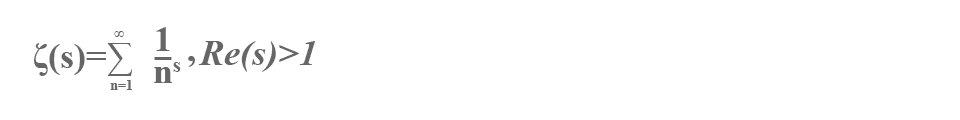This was problem #8 on Hilbert's list. There are many fine books on the zeta function and the Riemann hypothesis which are easy to locate. I leave the matter at this.

Problem 2 • The Poincare Conjecture

Suppose that a compact connected 3-dimensional manifold has the property that every circle in it can be deformed to a point. Then must it be homeomorphic to the 3-sphere?

The n-sphere is the spaceA compact n-dimensional manifold can be thought of as a closed bounded n-dimensional surface (di erentiable and non-singular) in some Euclidean space.

The n-dimensional Poincare conjecture asserts that a compact n-dimensional manifold M having the property that every map $f:{s}^{k}\to M$, $k\mathrm{<< /mi>n}$ (or equivalently, $k\le n/2$) can be deformed to a point, must be homeomorphic to ${s}^{n}$ .

Henri Poincaré studied these problems in his pioneering papers in topology. Poincare in 1900 (see Poincaré, 1953, pp 338{370) announced a proof of the general n-dimensional case. Subsequently (in 1904) he found a counter-example to his first version of the statement (Poincaré 1953, pp 435{498). In the second paper he limits himself to $n=3$, and states the 3-dimensional case as the problem above (not actually as a \conjecture").

My own relationship with this problem is described in the story (Smale, 1990a). There I wrote

I first heard of the Poincaré conjecture in 1955 in Ann Arbor at the time I was writing a thesis on a problem of topology. Just a short time later, I felt that I had found a proof (3 dimensions). Hans Samelson was in his office, and very excitedly I sketched my ideas to him. ... After leaving the office, I realized that my "proof" hadn't used any hypothesis on the 3-manifold.

In 1960, "on the beaches of Rio", I gave an armative answer to the n-dimensional Poincare conjecture for $n>4$. In 1982, Mike Freedman gave an affirmative answer for $n=4$. (Note: for $n>4$, I proved the stronger result that M was the smooth union of two balls, $M={D}^{n}\cup {D}^{n}$; that result is unproved for $n=4$, today.)

For background on these matters, besides the above references, see (Smale, 1963).

Many other mathematicians after Poincaré have claimed proofs of the 3-dimensional case. See (Taubes, 1987) for an account of some of these attempts.

A reason that Poincaré's conjecture is fundamental in the history of mathematics is that it helped give focus to a manifold as an object of study in its own right. In this way, Poincaré influenced much of 20th century mathematics with its attention to geometric objects including eventually algebraic varieties, Riemannian manifolds, etc.

I hold the conviction that there is a comparable phenomenon today in the notion of a "polynomial time algorithm". Algorithms are becoming worthy of analysis in their own right, not merely as a means to solve other problems. Thus I am suggesting that as the study of the set of solutions of an equation (e.g. a manifold) played such an important role in 20th century mathematics, the study of finding the solutions (e.g. an algorithm) may play an equally important role in the next century.

Problem 3 • Does P=NP?

I sometimes consider this problem as a gift to mathematics from computer science. It may be useful to put it into a form which looks more like traditional mathematics.

Towards this end first consider the Hilbert Nullstellensatz over the complex numbers. Thus let ${f}_{1}$ ,…, ${f}_{k}$ be complex polynomials in n variables; we are asked to decide if they have a common zero $\zeta \in {C}^{n}$. The Nullstellensatz asserts that this is not the case if and only if there are complex polynomials ${g}_{1}$,…, ${g}_{k}$ in n variables satisfying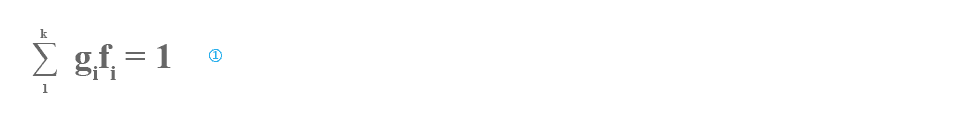as an identity of polynomials.

The effective Nullstellensatz as established by Brownawell (1987) and others, states that in , the degrees of the gi may be assumed to satisfy

$\mathrm{deg}{g}_{i}\le \mathrm{max}{\left(3,D\right)}^{n}$, $D=\mathrm{max}\mathrm{deg}{f}^{i}$.

With this degree bound the decidability problem becomes one of linear algebra. Given the coefficients of the ${f}_{i}$ one can check if has a solution whose unknowns are the coefficients of the ${g}_{i}$. Thus one has an algorithm to decide the Nullstellensatz. The number of arithmetic steps required grows exponentially in the number of coefficients of the ${f}_{i}$ (the input size).

Conjecture (over $C$ ). There is no polynomial time algorithm for deciding the Hilbert nullstellensatz over $C$ .

A polynomial time algorithm is one in which the number of arithmetic steps is bounded by a polynomial in the number of coefficients of the ${f}_{i}$, or in other words, is polynomially bounded.

To make mathematical sense of this conjecture, one has need of a formal definition of algorithm. In this context, the traditional definition of Turing machine makes no sense. In (Blum-Shub-Smale, 1989) a satisfactory satisfactory is proposed, and the associated theory is exposed in (Blum-Cucker-Shub-Smale (or BCSS), 1997).

Very briefly, a machine over $C$ has as inputs a nite string ( ... ${x}_{-1}$; ${x}_{0}$; ${x}_{1}$, ... ) of complex numbers and the same for states and outputs. Computations on states include arithmetic operations and shifts on the string. Finally, a branch operation on "${x}_{1}=0?$" is provided.

The size of an input is the number of elements in the input string. The time of a computation is the number of machine operations used in the passage from input to output. Thus a polynomial time algorithm over $C$ is well-defined.

Note that all that has been said about the machines and the conjecture use only the structure of $C$ as a field and hence both the machines and conjecture make sense over any field. In particular if the field is ${Z}_{2}$ of two elements, we have the Turing machines.

Consider the decision problem: Given (as input) $k$ polynomials in n variables with coefficients in ${Z}_{2}$. Decide if there is a common zero $\zeta \in {\left({Z}_{2}\right)}^{n}$?

This is a reformulation of the classic conjecture $P\ne NP$.

In the above we have bypassed the basic ideas and theorems related to NP-completeness. For the classic case of Cook and Karp, see (Garey-Johnson, 1979), and for the theory over an arbitrary field, see BCSS.

It is useful for some of the problems below (7,9,17,18) to have the definition of a machine over the real numbers $R$. In fact, the only change in the definition over $C$ , is to take the branch operation to be "${x}_{1}\le 0$?"

Remark: In his foreward to BCSS, Dick Karp writes that he is inclined to think that the complexity question over $C$ above and the classical P versus NP question are very different and need to be attacked independently. On the other hand just after our book went to press, I noticed that there was a strong connection between the old and the new theories. BPP denotes the set of problems that can be solved in polynomial time (classically) using randomization. It is in a certain practical sense, almost the same as the class P. By using a short argument, reduction of polynomial systems modulo a random prime, supported by a useful conversation with Manuel Blum, I saw that, not $NP\subset BPP$ (classic) implies $P\ne NP$ over $C$ , that is, the conjecture over $C$ above. This result is also implicit in (Cucker et al, 1995).

Problem 4 • Integer zeros of a polynomial of one variable

Let us start by defining a diophantine invariant $\tau$ motivated by complexity theory. A program for a polynomial $f\in Z\left[t\right]$ of one variable with integer coefficients is the object ($1$, $t$, ${u}_{1}$, ... , ${u}_{k}$) where ${u}_{k}=f$, and for all $l$, ${u}_{l}={u}_{i}\circ {u}_{j}$ , $i,j\mathrm{<< /mi>l}$, and$\circ$ is $+$ or $-$ or $×$. Here ${u}_{0}=t$, ${u}_{-1}=1$. Then $\tau \left(f\right)$ is the minimum of $k$ over all such programs.

Is the number of distinct integer zeros of $f$, polynomially bounded by $\tau \left(f\right)$?

In other words, is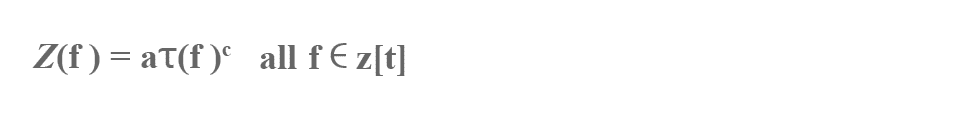Here $Z\left(f\right)$ is the number of distinct integer zeros of $f$ with $a$, $c$ universal constants.

From earlier results of Strassen, communicated via Sch¨onhage, Shub, and B¨urgisser, it follows that the exponent c has to be at least 2.

Mike Shub and I discovered this problem in our complexity studies. We proved that an affirmative answer implied the intractibility of the Nullstellensatz as a decision problem over $C$ and thus $P\ne NP$ over $C$ . See (Shub-Smale, 1995) and also BCSS.

Since the degree of $f$ is less than or equal to ${2}^{\tau }$ , $\tau =\tau \left(f\right)$, there are no more than ${2}^{\tau }$ zeros altogether.

For Chebyshev polynomials, the number of distinct real zeros grows exponentially with $\tau$ .

Many of the classic diophantine problems are in two or more variables. This problem asks for an estimate in just one variable, and nevertheless seems not so easy.

Here is a related problem. A program for an integer m is the object ($1$;${m}_{1}$, ... ,${m}_{l}$ ) where ${m}_{l}=m$, ${m}_{0}=1$, ${m}_{q}={m}_{o}\circ {m}_{j}$, $i,j\mathrm{<< /mi>q}$ and $\circ$ = $+$; $-$ or $×$. Then let $\tau \left(m\right)$ be the minimum of $l$, over all such programs. Thus $\tau \left(m\right)$ represents the shortest way to build up an integer $m$ starting from 1 using plus, minus, and times.

Problem: Is there a constant $c$ such that $\tau \left(k!\right)\le {\left(\mathrm{log}k\right)}^{c}$ for all integers $k$? One might expect this to be false, so that $k!$ is "hard to compute" (see Shub-Smale, 1995).

Problem 5 • Height bounds for diophantine curves

Can one decide if a diophantine equation $f\left(x,y\right)=0$ (input $f\in Z\left[u,v\right]$) has an integer solution, $\left(x,y\right)$, in time ${2}^{s}{}^{c}$ where $c$ is a universal constant? That is, can the problem be decided in exponential time?

Here $s=s\left(f\right)$ is the size of $f$ defined by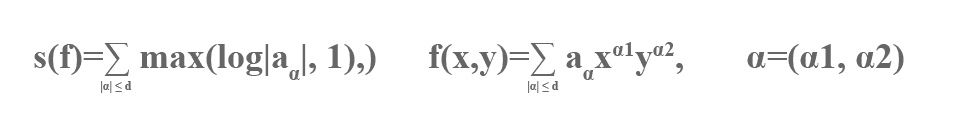and $\left|\alpha \right|={\alpha }_{1}+{\alpha }_{2}$, ${\alpha }_{i}\ge 0$.

The Turing model of computation is supposed, so “time”is the number of Turing operations.

This problem is essentially posed in (Cucker-Koiran-Smale, 1997), but it is a version of a well-known problem in number theory. The size $s\left(f\right)$ is a version of the “height" of $f$. Our problem is likely to be very dicult since it is not even known if one can decide this diophantine problem at all, let alone in exponential time. The solution of Hilbert's tenth problem by Matiyasevich (see Matiyasevich, 1993), using work of Davis, Robinson, Putnam shows the undecidability if the number of variables (27 is sucient if not 20 or even 11) is not restricted. A remaining important unsolved problem in this connection is:

Can one decide if there is a rational number solution to a given diophantine equation (any number of variables)?

The computer science notion of $NP$ is relevant to our main problem above. A problem in $NP$ is seen to be solvable in exponential time. The simple standard argument is given by noting that the test, evaluation of a polynomial, is done in polynomial time. Thus one might well ask the stronger question; is the two variable diophantine problem in $NP$?

To simplify the discussion we will now assume that the genus of the curve (defined by) $f$ is positive. The genus of a non-singular curve is the number of ”handles” in the homogenized curve of complex zeros. For a singular curve one can de ne the genus by taking an appropriate associated non-singular curve.

Consider the following hypothesis.

Height bound hypothesis: If the curve $f$, of positive genus, has any integer solution, then it has a solution $\left(a,b\right)$ satisfying the estimate; $\mathrm{log}\mathrm{max}\left(\left|a\right|,\left|b\right|\right)$ is polynomially bounded by $s\left(f\right)$.

For curves of positive genus, the height bound hypothesis implies that such a class of diophantine equations is in $NP$, and hence answers our main problem affirmatively.

One may ask how the height bound hypothesis relates to older conjectures in number theory. Thus let the strong height bound hypothesis be the strengthening of the height bound hypothesis to include all integer solutions. The Lang-Stark conjecture (see Lang, 1991), on certain curves of genus one, is implied by the strong height bound hypothesis, if one replaces our polynomially bounded by linearly bounded.

We don't claim real evidence for the height bound hypothesis. However there is in the background everywhere in this section, the Siegel theorem that there are only finitely many integer points on any diophantine curve of positive genus. A great challenge is to make Siegel's theorem effective; the height bound hypothesis is a version of such a goal.

For the case of genus one, and genus zero in case of a finite number of zeros, one does have the effectiveness results of Baker, and Baker-Coates, (see Baker, 1979), but even here they are somewhat weaker than the estimate of the strong height bound hypothesis.

The height bound hypothesis is false without the condition on the genus. This follows from the fact that the smallest integer solution of the "non-Pellian" equation ${x}^{2}-d{y}^{2}=-1$ can be very large relative to a family of $d$ (see Lagarias 1980). (Mazur (1994) points out a similar phenomenon for Pell's equation, conditional on the Gauss class number conjecture). Lagarias (1979) proves none the less that this equation (ie. the feasibility) is in $NP$ and moreover that the set of general binary quadratic equations is in $NP$. Manders-Adleman (1978) have shown some $NP$-completeness results on these problems.

There is also the more difficult version of all these problems when one uses the sparse representation of $f$, ie., in the de nition of $s\left(f\right)$ above delete the terms in which ${a}_{\alpha }=0$. Even the one variable case while true is not immediate. See (Cucker-Koiran-Smale, 1997), and (Lenstra, 1997).

Problem 6 • Finiteness of the number of relative equilibria in celestial mechanics

Is the number of relative equilibria nite, in the n-body problem of celestial mechanics, for any choice of positive real numbers ${m}_{1}$, ... , ${m}_{n}$ as the masses?

The problem is in Wintner's book (1941) on celestial mechanics. A relative equilibrium is a solution to Newton's equations which is induced by a plane rotation.

For the 3-body problem there are five relative equilibria: three found by Lagrange, two by Euler. There are “the Trojans” in the solar system, which correspond to the Lagrange relative equilibria. For 4-bodies the finiteness is unknown.

In (Smale, 1970), I interpreted the relative equilibria as critical points of a function induced by the potential of the planar $n$-body problem. More precisely the relative equilibria correspond to the critical points of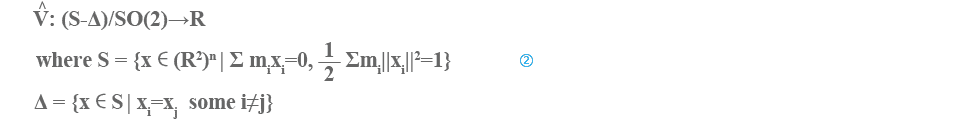The rotation group $SO\left(2\right)$ acts on $S-\Delta$ and is induced on the quotient from the potential function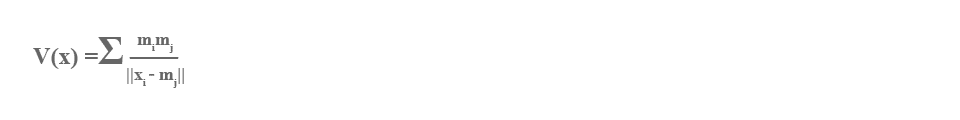Note that $V:S\to R$ is invariant under the rotation group $SO\left(2\right)$ and that the quotient space $S/SO\left(2\right)$ is homeomorphic to complex projective space of dimension $n-2$.

Thus the question has the equivalent form:

For any choice of ${m}_{1}$, ... , ${m}_{n}$ , does of $\left(2\right)$ have a finite number of critical points?

Mike Shub (1970) has shown that the set of critical points is compact.

Say that (${m}_{1}$ , ... , ${m}_{n}$ ) is critical if the corresponding has a degenerate critical point.

What is the nature of the set of critical masses in the n-dimensional spaces of masses? Does it have measure zero? or nite Betti numbers?

Palmore (1976) has shown that it is empty in case $n=3$ and not empty in case $n=4$. Kuz'mina, Moeckel, Xia, Albouy, and McCord, (see e.g. McCord, 1996), have further results on these problems.

G. D. Birkhoff asked the question: what is the topology of the constant angular momentum submanifolds of the $n$ body problem? In (Smale, 1970) I solved this problem for the case of $n$ bodies in the plane. See also (Easton, 1971). The case for n bodies in 3-dimensional space remains open: one obstacle is the solution of Wintner's problem above. However, recently, McCord-Meyer-Wang (1998), solved Birkhoff's problem for the 3 body problem in 3-space. See this paper also for a good historical and mathematical background for many of these things.

Further background may be found in (Abraham-Marsden, 1978).

Problem 7 • Distribution of points on the 2-sphere.

Let ${V}_{n}\left(x\right)={\Sigma }_{1\le i\mathrm{<< /mi>j\le N}}\mathrm{log}\genfrac{}{}{0.1ex}{}{1}{\left|\left|{x}_{i}-{x}_{j}\right|\right|}$ where $x=\left({x}_{1},...,{x}_{n}\right)$, the ${x}_{i}$ are distinct points on the 2-sphere ${S}^{2}\subset {R}^{3}$ , and $\left|\left|{x}_{i}-{x}_{j}\right|\right|$ is the distance in ${R}^{3}$. Denote $mi{n}_{x}{V}_{N}\left(x\right)$ by ${V}_{N}$

Can one find (x1 ,... , xN ) such that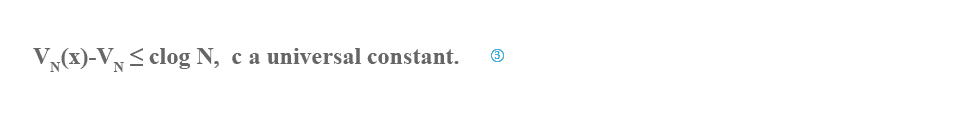For a precise version one could ask for a real number algorithm in the sense of BCSS which on input $N$ produces as output distinct ${x}_{1},...,{x}_{n}$ on the 2-sphere satisfying with halting time polynomial in $N$.

This problem emerged from complexity theory, jointly with Mike Shub (see Shub-Smale, 1993). It is motivated by finding a good starting polynomial for a homotopy algorithm for realizing the Fundamental Theorem of Algebra.

An $\left({x}_{1},...,{x}_{n}\right)=x$ such that ${V}_{N}\left(x\right)={V}_{N}$ is called an $N$-tuple of elliptic Fekete points (see Tsuji, 1959).

The function ${V}_{N}$ as a function of $N$ satisfies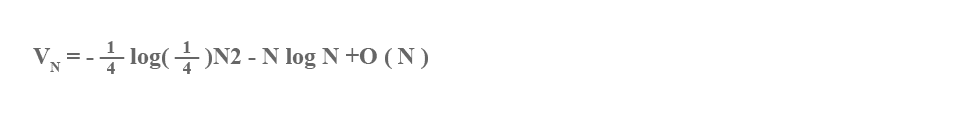It is natural also to consider the functions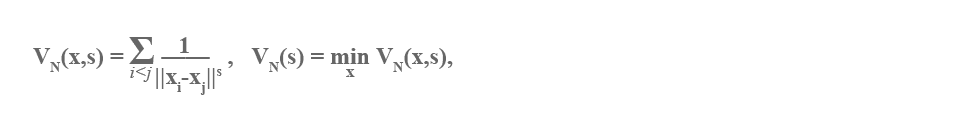$x$ as before and